# Example application

## Solt filling

RNN可以做智慧系统：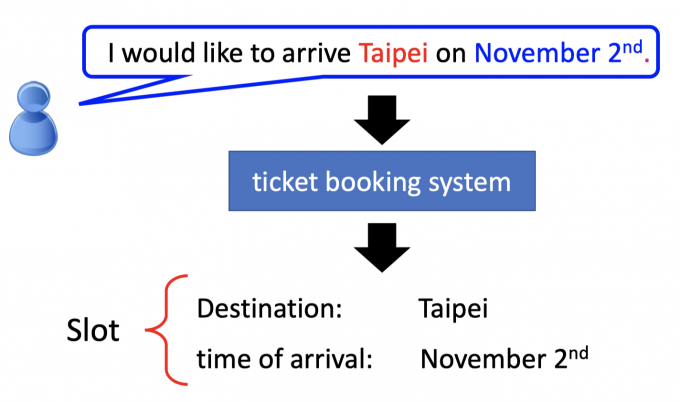## Encoding

How to represent each word as a vector?

### Beyond 1-of-N encoding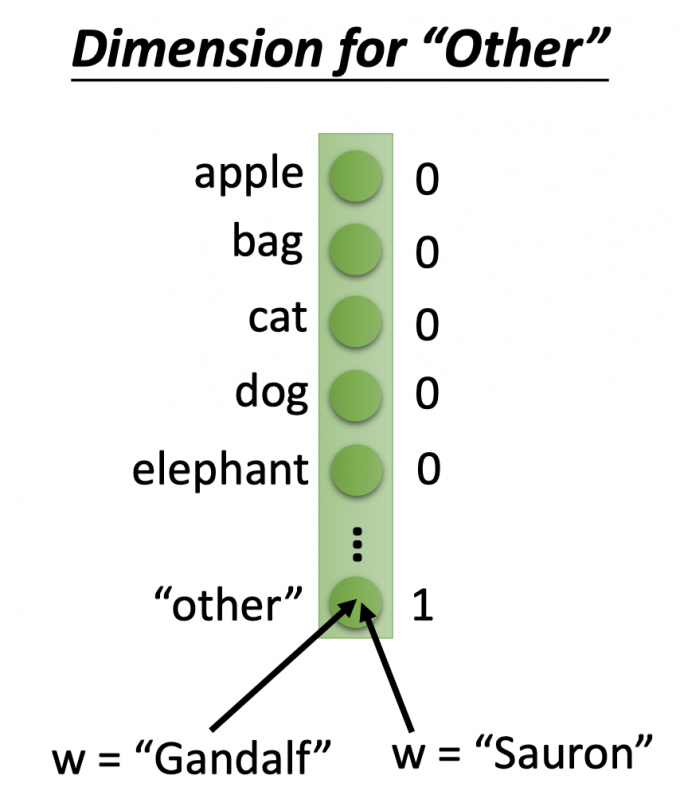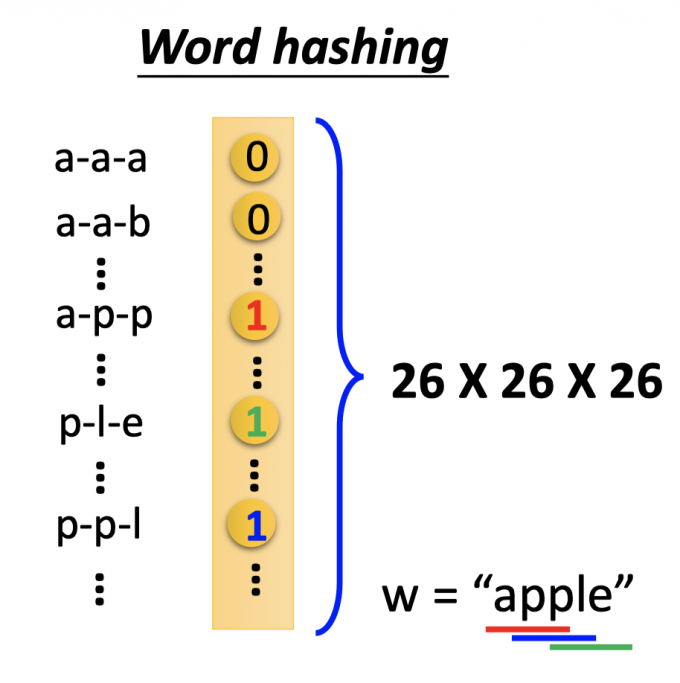### Example

input:一个单词（encoding为vector）

output: input单词中属于该槽位(solts)的概率分布(vector)。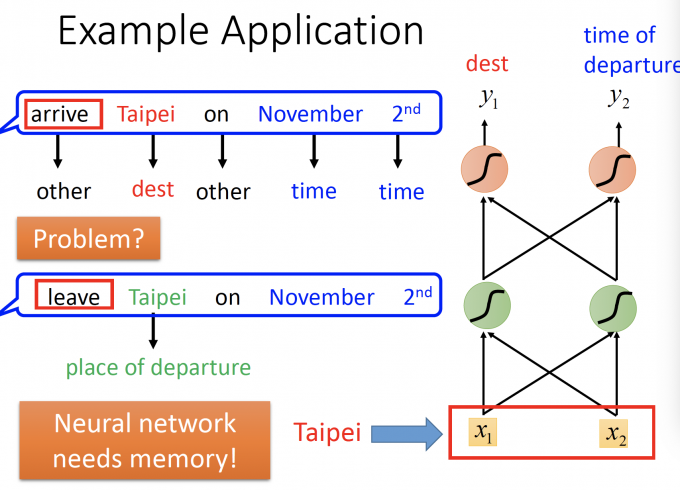# Recurrent Neural Network(RNN)

## Basic structure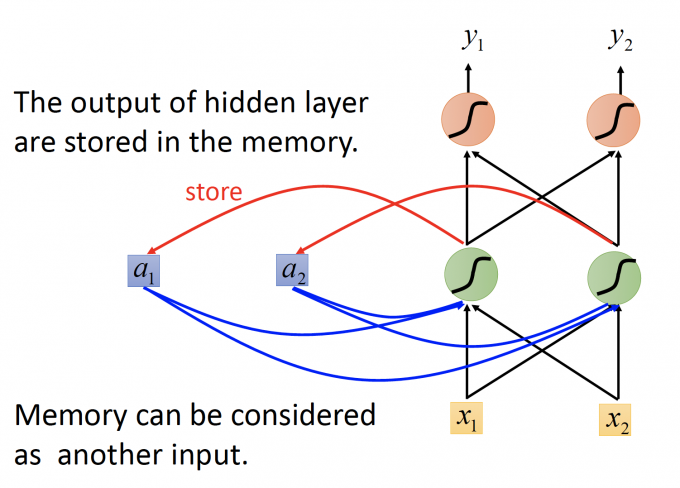Input sequence: $\begin{bmatrix}1 \ 1 \end{bmatrix}$ $\begin{bmatrix}1 \ 1 \end{bmatrix}$ $\begin{bmatrix}2 \ 2 \end{bmatrix}$ …

RNN模型如下图所示：所有的weight都是1，没有bias; 所有的神经元的activation function 都是线性的。

1. input : $\begin{bmatrix}1 \ 1 \end{bmatrix}$, 记忆元件初值 a1=0 a2=0.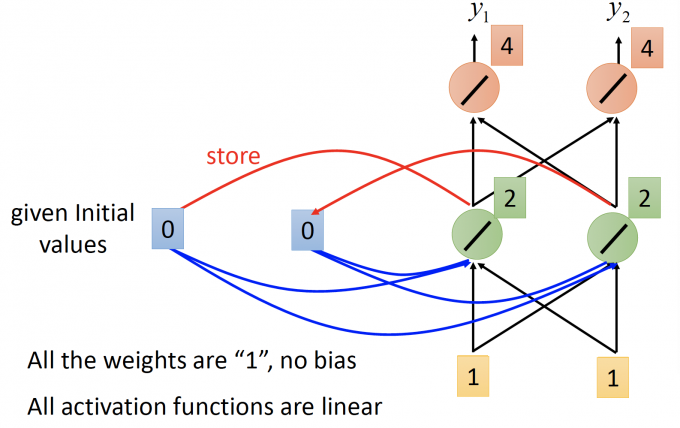记忆元件也作为输入的一部分，hidden layer的输出为 2 2, 更新记忆元件的值.

output: $\begin{bmatrix}4 \ 4 \end{bmatrix}$ , 记忆元件存储值 a1=2 a2=2.

2. input : $\begin{bmatrix}1 \ 1 \end{bmatrix}$ , 记忆元件存储值 a1=2 a2=2.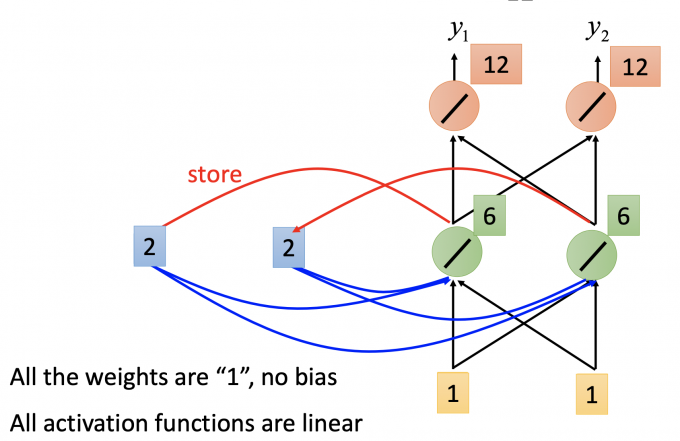output: $\begin{bmatrix}12 \ 12 \end{bmatrix}$ , 记忆元件存储值 a1=6 a2=6.

1. input : $\begin{bmatrix}2 \ 2 \end{bmatrix}$ , 记忆元件存储值 a1=6 a2=6.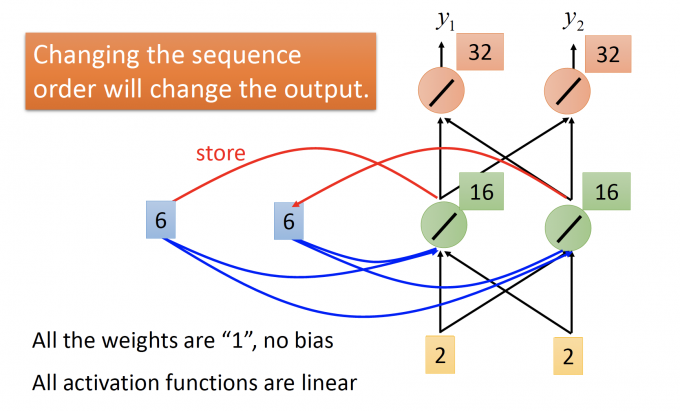记忆元件也作为输入的一部分，hidden layer 的输出为16 16,更新记忆元件的值。

output: $\begin{bmatrix}32 \ 32 \end{bmatrix}$ , 记忆元件存储值 a1=16 a2=16.

RNN中，由于有memory，会和一般前馈模型有两个不同的地方：一是输入相同的vector，输出可能是不同的；二是将一个sequence连续放进RNN模型中，如果sequence中改变顺序，输出也大多不同。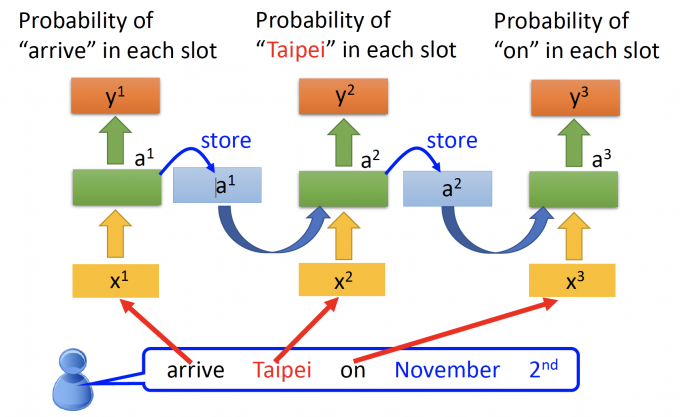## Elman Network & Jordan Network

（据说，记忆元件中存储output的值会有较好的performance，因为output是有target vector的，因此能具象的体现放进memory的是什么）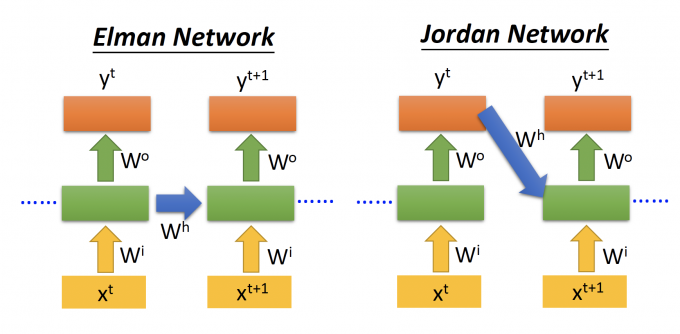## Bidirectional RNN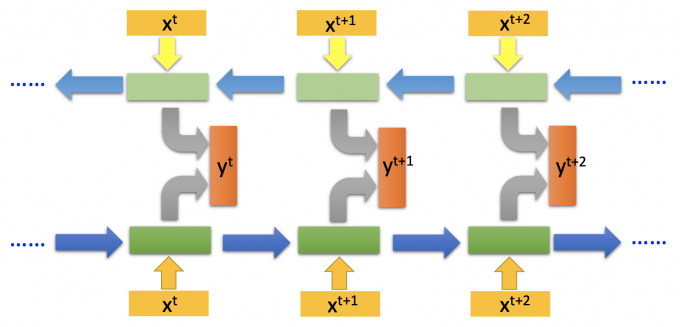## Long Short-term Memory(LSTM)

LSTM如下图，与一般NN不同的地方是，他有4个inputs,一个outputs。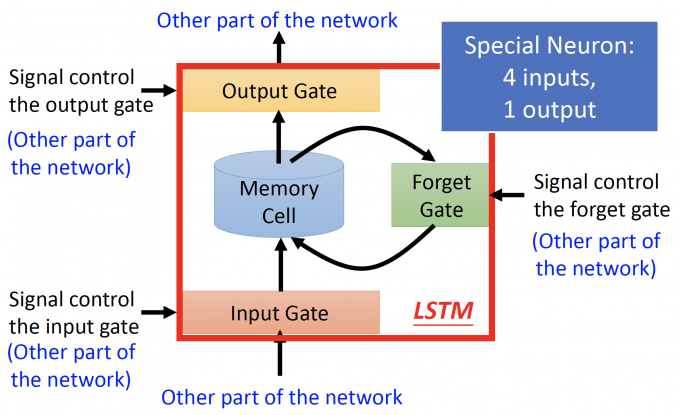LSTM主要有四部分组成：

• Input Gate：输入门，下方箭头是输入，左方箭头是输入信号控制输入门的打开程度，完全打开LSTM才能将输入值完全读入，打开的程度也是NN自己学。
• Output Gate：输出门，上方箭头是输出，左方箭头是输入信号控制输出门的打开程度，同理，打开程度也是NN自己学习。
• Memory Cell：记忆元件。
• Forget Gate：遗忘门，右边的箭头是输入信号控制遗忘门的打开程度，控制将memory cell洗掉的程度。

# LSTM

• $z_o,z_i,z_f$ 是门的signal control,其实就等同于一般NN中neuron的输入z，是scalar。
• gate其实就是一个neuron，通常gate neuron 的activation function f取 sigmod,因为值域在0到1之间，即对应门的打开程度。
• input/forget/output gate的neuron的activation function是f(sigmod function), input neuron的activation function是g。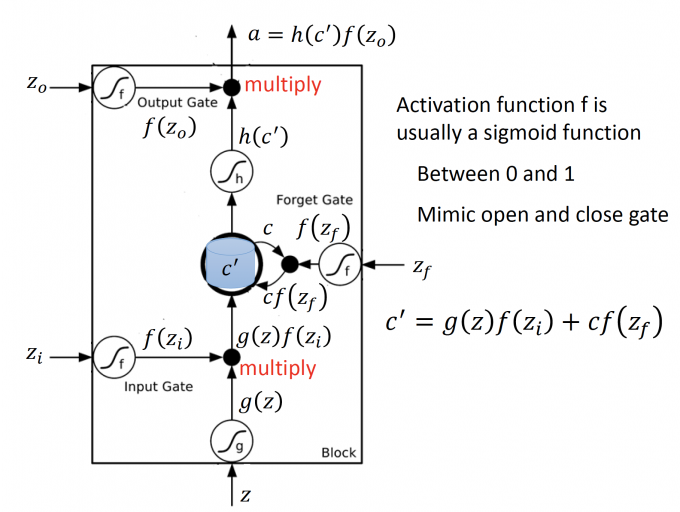1. input gate控制输入:$g(z)f(z_i)$
• input: z $\rightarrow$ $g(z)$
• input gate signal control: $z_i \rightarrow f(z_i)$
• multiply：$g(z)f(z_i)$
2. forget gate 控制memory：$cf(z_f)$
• forget gate signal control: $z_f\rightarrow f(z_f)$
• 如果 $f(z_f)=1$ ,说明memory里的值保留；如果 $f(z_f)=0$ ,说明memory里的值洗掉。
3. 更新当前时间点的memory(输入+旧的memory值) ：$c’=g(z)f(z_i)+cf(z_f)$
4. output gate 控制输出：$h(c’)f(z_o)$
• output: $c’ \rightarrow h(c’)$
• output gare signal control: $z_o \rightarrow f(z_o)$
• multiply: $h(c’)f(z_o)$

LSTM模型（trained）如下图：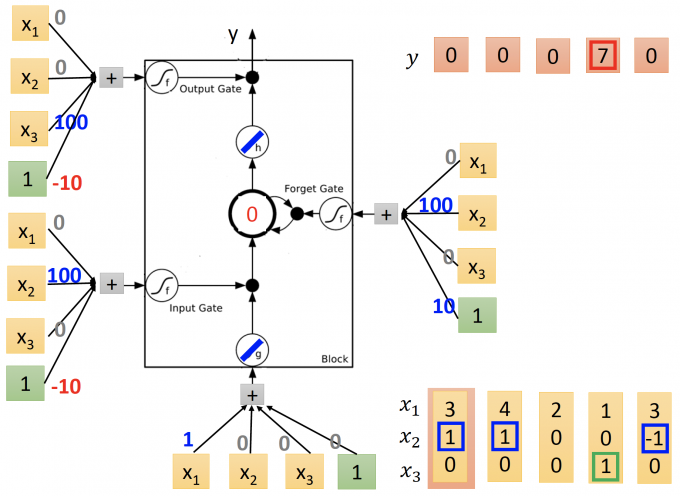（建议手算一遍）

## Compared with Original Network

original network如下图：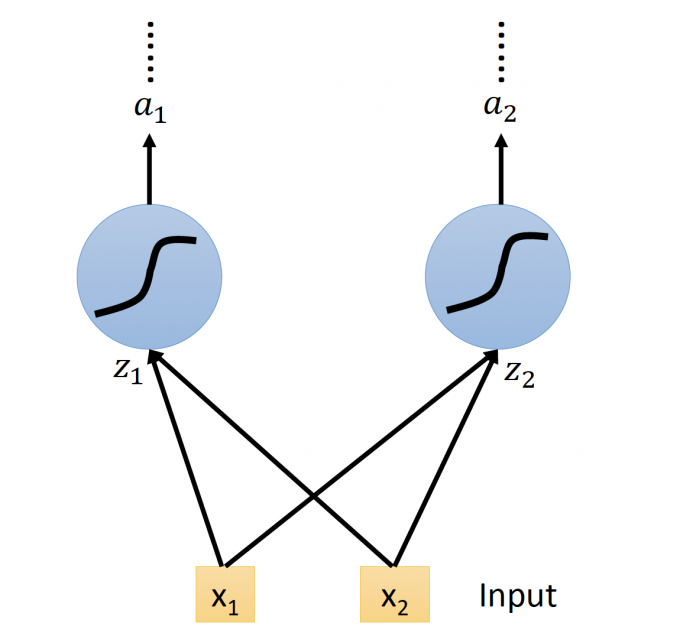LSTM 的NN即用LSTM替换原来的neuron，这个neuron有四个inputs，相对于original network也有4倍的参数，如下图：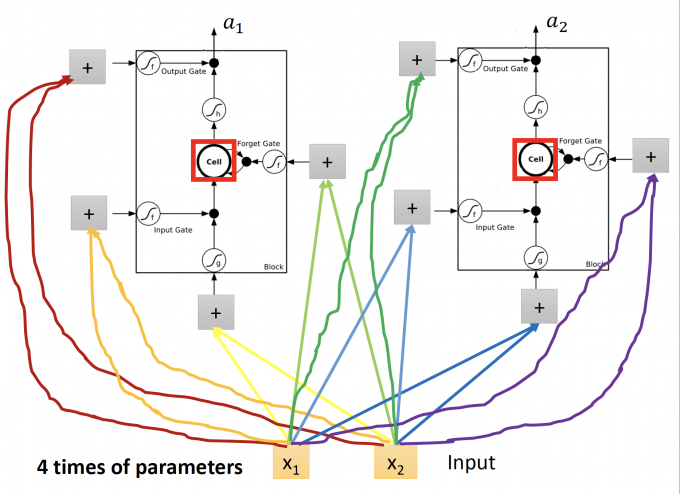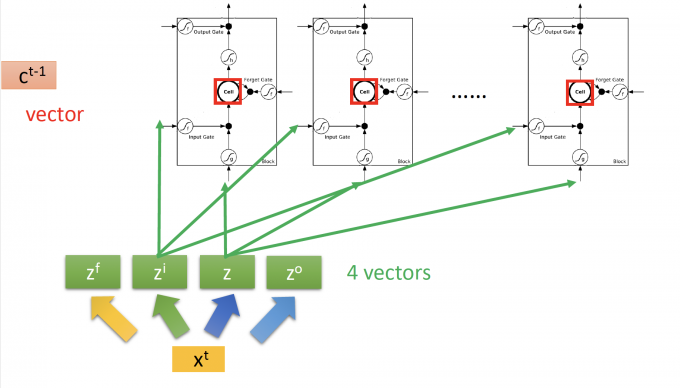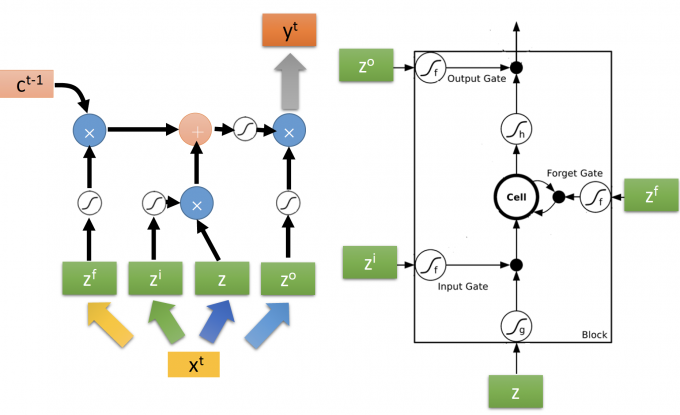## Extension：“peephole”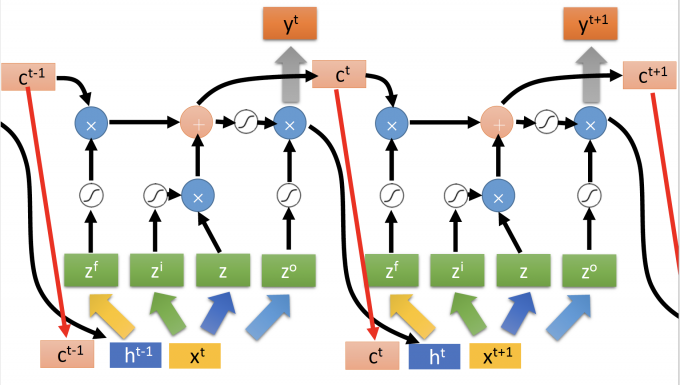## Multi-layer LSTM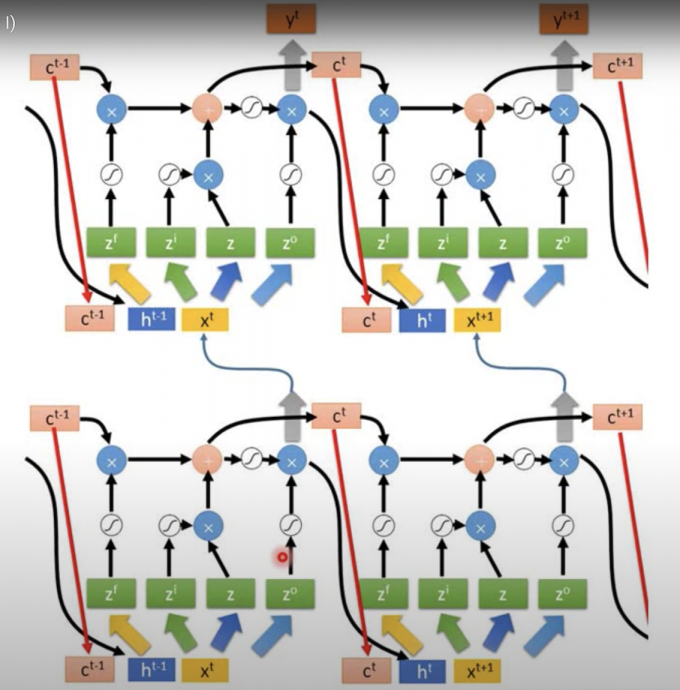（：wtf 我到底看到了什么

## Learning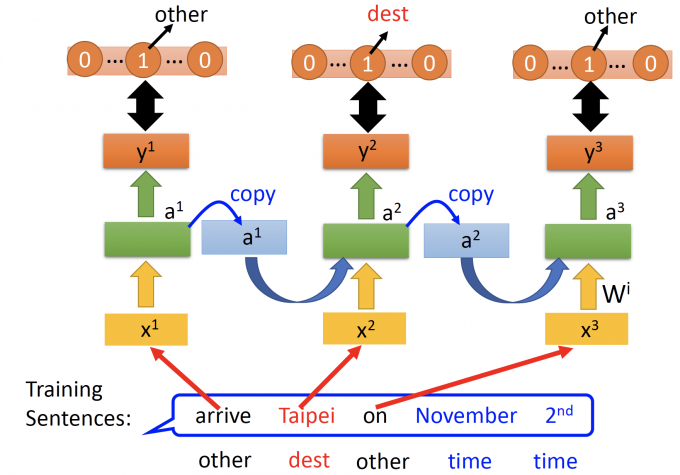RNN一般就用BPTT训练。

## How to train well

### not easy to train

RNN-based network is not always easy to learn.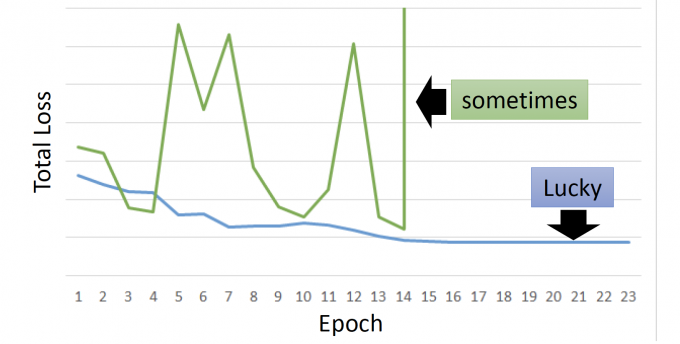### error surface is rough

error surface，即total loss在参数变化时的函数图。• 橙色点出发：

• 起初处在flat的位置。
• 可能稍微不幸，就会出现跨过悬崖，即出现了剧烈震荡的问题。

• 这样处在悬崖低的橙色点，（Clipping路线），更新就会到绿色的，继续更新。

### Why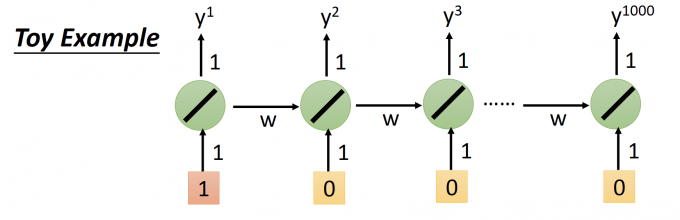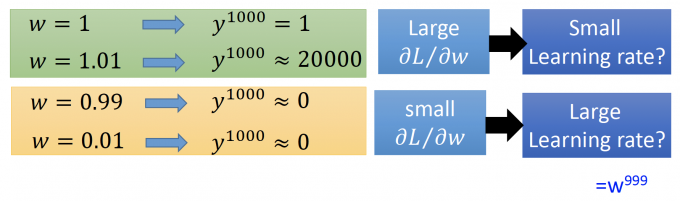• 绿色部分：当w从1变化为1.01时， $y^{1000}$ 的输出变化即大，既有较大的gradient，理应有小的learning rate。
• 黄色部分：当w从0.99变化为0.01时， $y^{1000}$ 的输出几乎不变化，即有较小的gradient，理应有大大learning rate.

Reason：RNN，虽然可以看作不同时间点的展开计算，但始终是同一个NN的权重计算（cell连接到下一个时间点的权重），在不同时间中，反复叠乘，因此会出现这种情况。

1. LSTM几乎已经算RNN的一个标准了，为什么LSTM的performance比较好呢。
• 为什么用LSTM替换为RNN？

: The influence never disappears unless forget gate is closed.（memory的影响可以很持久）

1. GRU（Gated Recurrent Unit）：是只有两个Gate，比LSTM简单，参数更少，不容易overfitting

2. 玄学了叭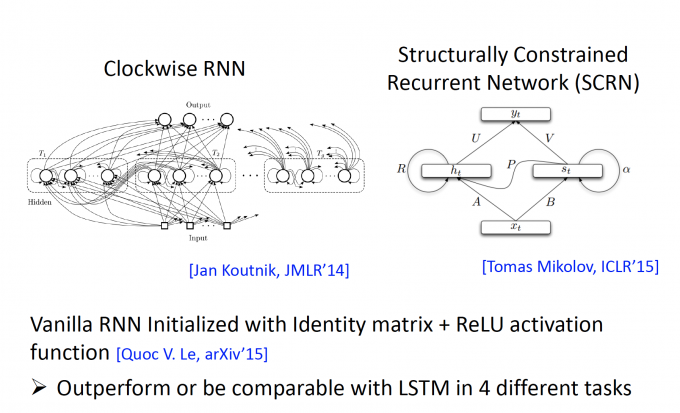【待更新】

1. BPTT
2. GRU